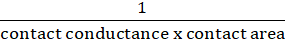1. Engineering
2. Mechanical Engineering
3. a pipe is constructed of two cylindrical shells joined by...

# Question: a pipe is constructed of two cylindrical shells joined by...

###### Question details
1. A pipe is constructed of two cylindrical shells joined by a shrink fit, as shown in the figure. The inner shell is stainless steel with a thermal conductivity of 15.1 W/m∙K and a thickness of 3 mm. The outer shell is Inconel with a thermal conductivity of 11.6 W/m∙K and a thickness of 2.5 mm. The contact conductance between the Inconel and the stainless is 4310 W/m2K. (Note that in this case there is an extra thermal resistance term associated with the contact, and Rcontact= 1/contact conductance x contact area). A liquid solution flows inside the pipe with a heat transfer coefficient of 3200 W/m2K and water flows over the exterior of the pipe with heat transfer coefficient of 7900 W/m2K.
1. Calculate the total thermal resistance from the liquid solution to the water for a pipe 1 meter long. Assume no fouling and use dimensions on the figure.
2. What is the controlling resistance?###### Solution by an expert tutor